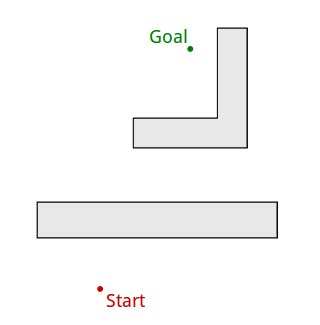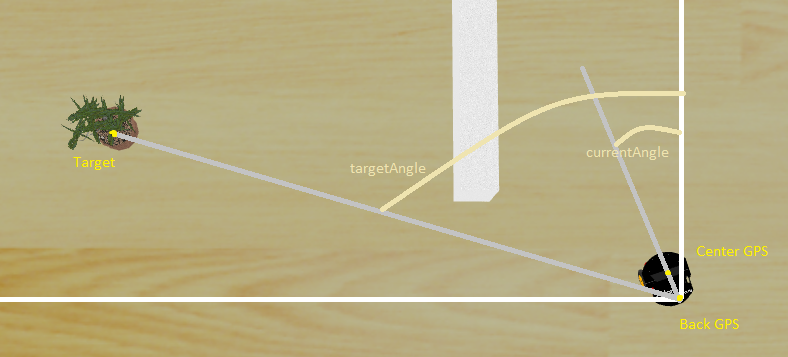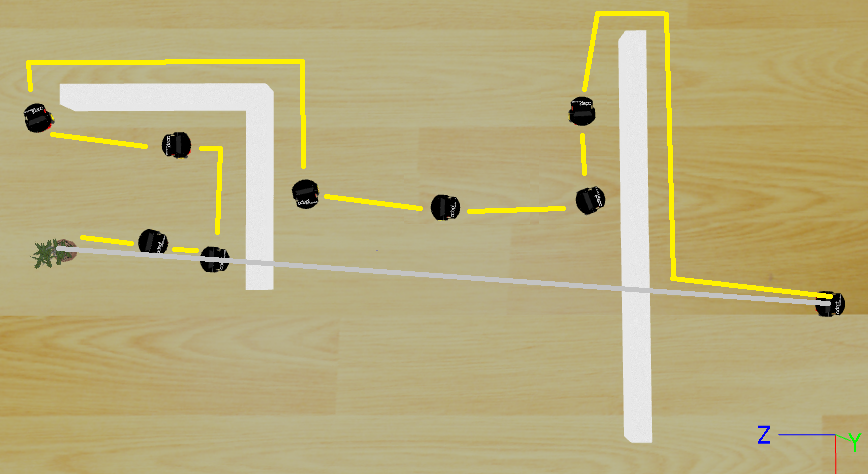#### Can Güney Aksakalli

Notes from my journey

This article aims to explain implementation of bug motion planning algorithms in Webots robot simulation environment.

Webots is a robot simulation environment widely used for educational purpose. You can edit environment with its GUI and write controller program for mobile robots in C, C++, Java, Python and MATLAB. It is very convenient tool to work on robotic algorithms, but it is not free. Fortunately, I had Webots EDU license to work with this tool.

The robot knows the distance to goal and the direction. Here is the plan of the environment:I used a pioneer robot equipped with following sensors:

• 2x GPS
• 7x infra-red front side sensor
• 1x Kinect

The algorithms were implemented in three individual projects with the same sensors. These motion planning algorithms’ goal is to reach the target. Ported tree represents the target in the simulation environment.

Kinect detects the obstacle and the robot turns right or left to avoid it. It starts to move around this obstacle and the infra-red sensors control the distance through the obstacle.

Two GPS sensors are located in the robot to determine the position and angle of the target. Back GPS to center GPS angle is defined as “currentAngle”, back GPS to target is defined as “targetAngle”. (Although the accuracy of GPS wouldn’t allow to determine this in real world, it was a quite good approach for this environment I believe.)When the robot in “GO_TO_TARGET” state, it tries to reduce the difference of two angles. When the target angle is bigger than current angle; speed of right wheel is increased and speed of left wheel is decreased. It is vice versa when the target angle is smaller than current angle. This progress continues up to threshold value. If the difference of two angles is smaller than that value, both wheels are set to normal speed.

## Bug0 Algorithm

• Continue

It was not defined as a monotonically right or left turning algorithm so the pioneer robot turns in direction that has longer distance to the wall. It follows the wall until the side sensors not detect an obstacle and then it tries to go the target. When it reaches the target, the main while loop is over and the robot stops.

## Bug1 Algorithm

• If an obstacle is encountered, circumnavigate it and remember how close you get to the goal

The Robot has two states

• GO_TO_TARGET : The robot tries to go to target point with calculating the angles. If it detects an obstacle stores the initial minimum distance values that coordinate point, time and distance to target.
• FOLLOW_OBSTACLE : The robot follows the obstacle and stores minimum distance point for its route. When it is at the closest point for second time, it switches to “GO_TO_TARGET” state. How can the robot understand that this is first or second time that it passes through the minimum distance point? The robot also saves the time period of reaching the minimum point. It is assumed that this robot can not turn around a obstacle less than 3 seconds. If there is more than three seconds differences between reaching the short distance point, it must be second time. Otherwise, it would always switches to “GO_TO_TARGET” state because it reaches the smallest point for every step during coming closer to target.

## Bug2 Algorithm

• Head toward goal on the m-line
• If an obstacle is in the way, follow it until you encounter the m-line again closer to the goal.
• Leave the obstacle and continue toward the goal

The robot calculates its initial angle to target. In “GO_TO_TARGET” state, it tries to reach the target. If it detects an obstacle, it switches to “TURN_RIGHT_FOLLOW” or “TURN_LEFT_FOLLOW” state. Actually they are making the same things accept turning directions. In following state, the robot follows the obstacle until reaching the same angle that in the initial step.Here is the code:

References

• Howie Choset, Robotic Motion Planning: Bug Algorithms [lecture slides].